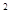• 计算(−)2008×0.82009得(     )

A.0.8
B.-0.8
C.+1
D.-1
单选题|常考题|普通|组卷 0

• 计算(－a2)3的结果是

A.a5
B.－a5
C.a6
D.－a6
单选题|常考题|容易|组卷 0
• 下列计算正确的是（    ）

A.B.C.D.单选题|模拟题|普通|组卷 0
• 下列运算正确的是(         )
A.a+a=aB.a·a=aC.(ab)=a·bD.(a)=a单选题|常考题|普通|组卷 1 |
• 下列计算正确的是(   )
A.2a+3b=5ab
B.=1
C.D.单选题|常考题|普通|组卷 0 |
• 下列运算正确的是（    ）

A.a3a3=a
B.C.D.单选题|常考题|普通|组卷 2
• 下列计算正确的是（　　）

A.a3+a2=2a5
B.（﹣2a32=4a6
C.（a+b）2=a2+b2
D.a6÷a2=a3
单选题|常考题|普通|组卷 0
• 下列运算结果正确的是（     ）
A.B.C.D.单选题|常考题|普通|组卷 0 |
• 判断312是96的几倍？（  ）

A.1
B.()2
C.()6
D.(－6)2
单选题|常考题|普通|组卷 0
• 计算3n•（　　）=﹣9n+1，则括号内应填入的式子为（　　）

A.3n+1
B.3n+2
C.﹣3n+2
D.﹣3n+1
单选题|常考题|普通|组卷 0
• 计算a3   ·  a2的结果是 （   ）

A.a6
B.a5
C.a
D.a9
单选题|模拟题|普通|组卷 0

0

TOP